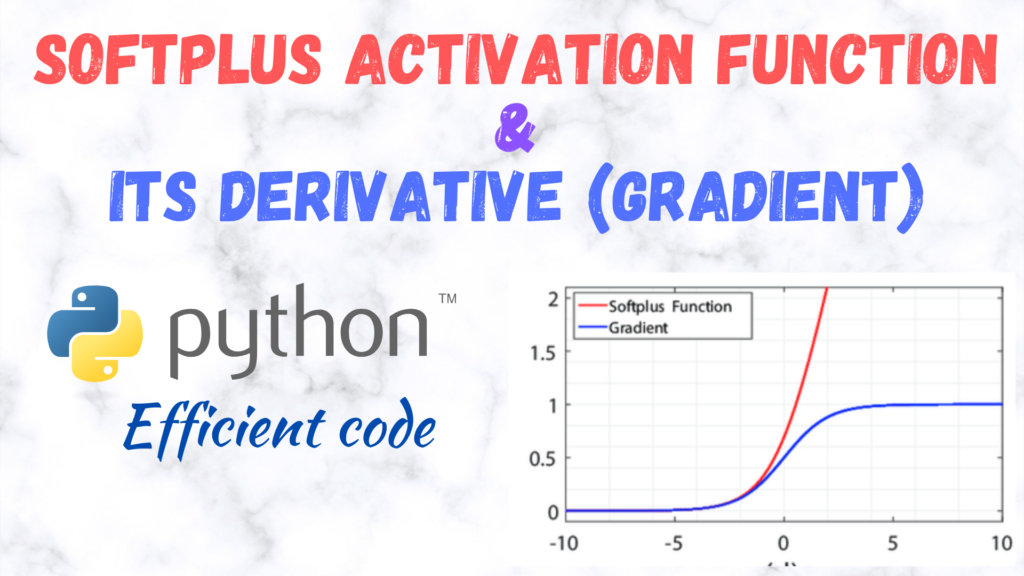# Efficient implementation of Softplus activation function and its Derivative (gradient) in PythonThe mathematical definition of the Softplus activation function is$f(x)=\log \left(1+e^{x}\right)$

with the derivative defined as$f^{\prime}(x)=\frac{1}{1+e^{-x}}$, which is actually the Sigmoid function. We have already discussed some efficient and stable implementations of the Sigmoid function here.

The Softplus function and its derivative for a batch of inputs (a 2D array with nRows=nSamples and nColumns=nNodes) can be implemented in the following manner:
Softplus simplest implementation

import numpy as np
def Softplus(x):
return np.log(1 + np.exp(-np.abs(x))) + np.maximum(x,0)


oftplus gradient simplest implementation

import numpy as np
return np.divide(1.,1.+np.exp(-x))



The above implementations are not stable enough, however, and can result in over/underflow (Reference: https://stackoverflow.com/questions/44230635/avoid-overflow-with-softplus-function-in-python)

The following is a stable implementation of the Softplus function

def Softplus(x):
# Reference: https://stackoverflow.com/questions/44230635/avoid-overflow-with-softplus-function-in-python
return np.log1p(np.exp(-np.abs(x))) + np.maximum(x, 0)
# return np.log(1 + np.exp(-np.abs(x))) + np.maximum(x,0)


For the gradient of the Softplus function, we can make use of the stable and efficient implementation of the Sigmoid function, described here.

def Sigmoid(x): # Also known as logistic/soft step or even expit in scipy.special
# Alternative 1 (Doesn't work with Numba as boolean masking (fancy indexing) is not supported for 2D arrays -https://stackoverflow.com/questions/57915632/numba-nopython-mode-cannot-accept-2-d-boolean-indexing )
# Hao Peng's answer from here https://stackoverflow.com/questions/51976461/optimal-way-of-defining-a-numerically-stable-sigmoid-function-for-a-list-in-pyth
pos_mask = (x >= 0)
# Boolean array inversion is faster than another comparison
z = np.zeros_like(x)
top = np.ones_like(x)

def Softplus_grad(x): # This is simply the sigmoid function
return Sigmoid(x)


Please note, and I can’t stress this enough, the above and the following implementations are only tested and fine-tuned for a batch of inputs, i.e., the expected input for the functions is a 2d array with rows representation different samples, and columns representing different nodes.

Furthermore, these implementations can still be accelerated (sped-up) by using Numba (https://numba.pydata.org/). Numba is a Just-in-time (JIT) compiler that

translates a subset of Python and NumPy code into fast machine code.

To use numba, install it as:

pip install numba


Also, make sure that your numpy is compatible with Numba or not, although usually pip takes care of that. You can get the info here: https://pypi.org/project/numba/

Accelerating the above functions using Numba is quite simple. Just modify them in the following manner:

Softplus NUMBA implementation

from numba import njit
@njit(cache=True,fastmath=True)
def Softplus(x):
# Reference: https://stackoverflow.com/questions/44230635/avoid-overflow-with-softplus-function-in-python
return np.log1p(np.exp(-np.abs(x))) + np.maximum(x, 0)
# np.log(1 + np.exp(-np.abs(x))) + np.maximum(x,0)



Softplus derivative (gradient) NUMBA implementation

from numba import njit

@njit(cache=True,fastmath=True, parallel=True)
def Sigmoid(x): # Also known as logistic/soft step or even expit in scipy.special
# Hao Peng's answer from here https://stackoverflow.com/questions/51976461/optimal-way-of-defining-a-numerically-stable-sigmoid-function-for-a-list-in-pyth
# Works only for 2D arrays
output = np.zeros((x.shape,x.shape),dtype=x.dtype)
for i in prange(x.shape):
for j in range(x.shape):
x_val = x[i,j]
if x_val>=0:
output[i,j] = 1. / ( 1. + np.exp(-x_val) )
else:
e_x = np.exp(x_val)
output[i,j] = e_x / ( 1. + e_x )
return output

@njit(cache=True,fastmath=True)
def Softplus_grad(x): # This is simply the sigmoid function
# The following would be susceptible to over/underflow just like th Sigmoid function
# return np.divide(1.,1.+np.exp(-x))
# Use this instead
return Sigmoid(x)


This is quite fast and competitive with Tensorflow and PyTorch (https://github.com/manassharma07/crysx_nn/blob/main/benchmarks_tests/Performance_Activation_Functions_CPU.ipynb).

It is in fact also used in the CrysX-Neural Network library (crysx_nn)

Moreover, the above implementations can be further accelerated using Cupy (CUDA), if using single precision (float32) is not a problem.

CuPy is an open-source array library for GPU-accelerated computing with Python. CuPy utilizes CUDA Toolkit libraries to make full use of the GPU architecture.

The Cupy implementations look as follows:

import cupy as cp
def Softplus_cupy(x):
# Reference: https://stackoverflow.com/questions/44230635/avoid-overflow-with-softplus-function-in-python
return cp.log1p(cp.exp(-cp.abs(x))) + cp.maximum(x, 0)
# np.log(1 + np.exp(-np.abs(x))) + np.maximum(x,0)


def Sigmoid_cupy(x):

def Softplus_grad_cupy(x): # This is simply the sigmoid function
# The following would be susceptible to over/underflow just like th Sigmoid function
# return cp.divide(1.,1.+cp.exp(-x))
# Use this instead
return Sigmoid_cupy(x)


The above code is also used in the crysx_nn library.
To see how the crysx_nn implementations of Softplus compare with TensorFlow and PyTorch, click here.

I hope you found this information useful.

If you did, then don’t forget to check out my other posts on Machine Learning and efficient implementations of activation/loss functions in Python.[wpedon id="7041" align="center"]